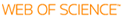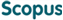### BROWSE

On Boundary Layers for the Viscous Burgers Equations in Various Domains

Cited 0 times inCited 0 times inTitle
On Boundary Layers for the Viscous Burgers Equations in Various Domains
Author
Issue Date
2020-02
Publisher
Abstract
The viscous Burgers equation is a well-known nonlinear equation for being studied before studying the Navier -Stokes equations. Here, we analyse layers for the viscous Burgers equation in various bounded domains with different boundary data instead of analysing those for the Navier-Stokes equations. There are two kinds of the layers for the viscous Burgers equation: One is interior layers inside the domain. Another is Boundary layers near the boundaries. In addition, the shapes of the layers change as the shapes of boundaries are different. However one thing is common that the layers are observed to get singularly steeper proportional to 1/ε as the viscous coefficient ε becomes smaller. In this thesis, we propose each layer corrector equations corresponding to each kind of layers and to each different domain such as a bounded line, a whole line in R, and a circle in R^2 , to approximate the steep layers. After that, we define the asymptotic expansions of the ε with the correctors and fully prove the convergence rate of the expansions to the viscous solution in terms of ε in L^2. Finally, we isualize the validation of the expansions using numerical experiments.
Description
Department of Mathematical Sciences
URI